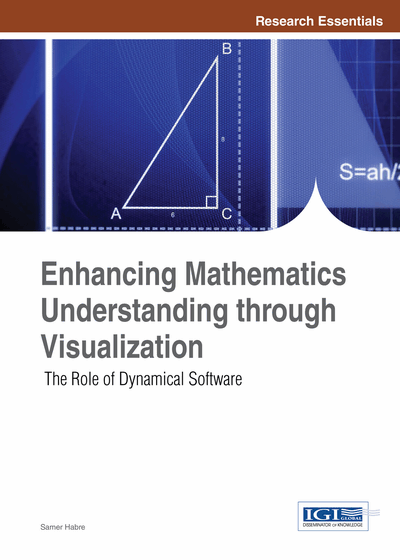# Dynamical Mathematical Software: Tools for Learning and for Research

Samer Habre (Lebanese American University, Lebanon)
DOI: 10.4018/978-1-4666-4050-4.ch004
Available
\$37.50
No Current Special Offers

## Abstract

Understanding mathematical concepts is many-folded. Traditional mathematics mostly emphasizes the algebraic/analytical aspect of a problem with minimal reference to its graphical aspect and/or numerical one. In a modern learning environment, however, multiple representations of concepts are proving to be essential for the teaching of mathematics. The availability of user-friendly dynamical software programs has paved the way for a radical yet smooth way for changing the way mathematical concepts are perceived. This chapter presents some of the author’s attempts for employing innovative methods in teaching topics in calculus, in differential and difference equations. The focus is on the use of dynamical programs that boost the visual component of the topics being investigated, hence contributing to a more complete understanding of these topics.
Chapter Preview
Top

## Introduction

In the year 2000, the Standards of the National Council of Teachers of Mathematics (NCTM, 2000) acknowledged that multiple representations help students solve problems, support their understanding of concepts, and better communicate mathematical ideas. Consequently, mathematical visualization has become a core element for learning mathematical concepts. The advancement of technology has largely reshaped the teaching and learning of mathematics. It is now widely accepted that the strength of technology is mainly in its capability for providing greater and easier access to multiple representations of concepts (Kaput, 1992; Porzio, 1999), in particular visualizing mathematical concepts and ideas, and even sometimes abstract theorems (Pool, 1992).This chapter is intended on one hand to present some ways to exploit the dynamical feature of few mathematical programs so as to introduce and clarify concepts in calculus and in an introductory differential equations course. On the other hand, dynamical programs can also be used to conjecture and write proofs and thus may also be useful for research purposes. The chapter ends with an example showing how a dynamical software program created for a specific purpose can help conjecture new results for linear iterative systems.

## Complete Chapter List

Search this Book:
Reset